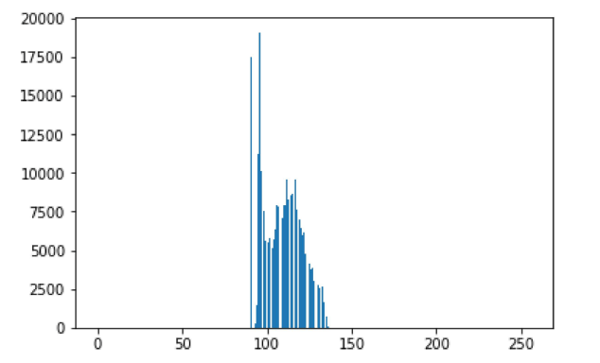Related Tags

python
communitycreator

# How to plot the histogram of an image in Python

Eman Kashif

Digital Image Processing is a significant aspect of data science. It is used in image modification and enhancement so we can acquire image attributes that lead to a greater understanding of data.

An image is made up of elements called pixels; the smallest pieces of information.

Pixels are arranged in a two-dimensional manner and are represented using squares.

### Properties

An image histogram is a representation of the range of tonal values that is present in an image.

The y-axis represents the gray level intensities, while the x-axis represents the frequency that corresponds to those respective intensities.

The x-axis depends on the number of bits used to store the image.

If 8 bits are used, the range will be from 0 to 255, making up a total of 256 intensity levels. This is usually the case with modern-day images.

#### Attributes of an image

We can determine a multitude of attributes of an image by looking at its histogram. If the bars are concentrated towards the left side of the histogram, it means that the image is on the darker side. Likewise, bars concentrated on the right side mean that the image is lighter.

#### Contrast of an image

Another attribute we can determine is the overall contrast of the image. If the bars are closer together over a low range of pixel values, the image contrast is low. If they are spread out over the x-axis, the contrast is high. The histogram below depicts a low contrast image:A histogram of an image is plotted by making two lists:

1. one containing the intensity levels
2. the other contains the frequency corresponding to those intensity levels

The matplotlib library is used to plot the histogram.

### Code example

Let’s look at the following code snippet:

#import libraries
import PIL
from PIL import Image
import matplotlib.pyplot as plt

#function to plot histogram
def histogram(img):
pixels=[]

#create list of values 0-255
for x in range(256):
pixels.append(x)

#initialize width and height of image
width,height=img.size
counts=[]

#for each intensity value
for i in pixels:
#set counter to 0
temp=0

#traverse through the pixels
for x in range(width):
for y in range(height):

#if pixel intensity equal to intensity level
#increment counter
if (img.getpixel((x,y))==i):
temp=temp+1

#append frequency of intensity level
counts.append(temp)

#plot histogram
plt.bar(pixels,counts)
plt.show()

In the example above, we have a function named histogram(img). This function takes an image as a parameter and plots a histogram for that image. It does so using the two lists corresponding to pixels (pixels) and the intensity (count) of those pixels.

RELATED TAGS

python
communitycreator

CONTRIBUTOR

Eman Kashif
RELATED COURSES

View all Courses

Keep Exploring

Learn in-demand tech skills in half the time